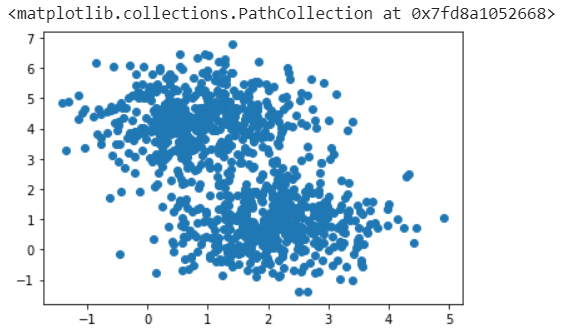# Keeping it simple

Original article was published by Ganesh Ravichandran on Deep Learning on MediumI have delved into learning about neural networks at least a dozen times, and learned a bit more each time. This time I want to start off with the simplest datasets and use them to understand network behavior. This article is the first in a series on my adventures with neural networks.

In this post, we are going to focus on multiclass classification. This is the problem of trying to identify which class a particular data point belongs to, from a limited set of classes.

The libraries we will use are `tensorflow` , `numpy` , `matplotlib`, and `sklearn` :

`import tensorflow as tfimport numpy as npimport matplotlib.pyplot as pltfrom sklearn.datasets import make_blobsfrom sklearn.model_selection import train_test_split`

We will start off by making two clusters of data:

`X, y = make_blobs(n_samples=1000, centers=2, n_features=2, random_state=0, cluster_std=0.9)y = tf.keras.utils.to_categorical(y)plt.scatter(X[:,0], X[:,1])`

Let’s split the data into training and test sets:

`X_train, X_test, y_train, y_test = train_test_split(X, y, test_size=0.33, random_state=42)`

Now for the actual model. We are going to build a multi-layer perceptron. The four layers will be Dense layers, with the last layer having a softmax activation function that will squash the predictions into a probability distribution that can be used to make a prediction between classes:

`model = tf.keras.Sequential()model.add(tf.keras.layers.Dense(16, input_shape=(2,), activation="relu"))model.add(tf.keras.layers.Dense(16, activation="relu"))model.add(tf.keras.layers.Dense(16, activation="relu"))model.add(tf.keras.layers.Dense(2, activation="softmax"))model.summary()`

Next is the model compilation step. We will use the Adam optimizer, and `categorical_crossentropy` to calculate loss in our multi-class classification problem. And we will measure the accuracy of our model as a metric.

`model.compile(    optimizer="adam",    loss="categorical_crossentropy",    metrics=["accuracy"])`

Let’s run 10 epochs:

`history = model.fit(    X_train,    y_train,    epochs=10,    validation_data=(X_test, y_test))`

Finally, we can take a look at the accuracy of the model on the training and testing data:

`_, train_accuracy = model.evaluate(X_train, y_train, verbose=0)_, test_accuracy = model.evaluate(X_test, y_test, verbose=0)print("Train: %.3f, Test: %.3f" % (train_accuracy, test_accuracy))>> Train: 0.957, Test: 0.948`

On the training data, our model has 95.7% accuracy, and 94.8% on the test data. This makes sense, since the model has seen the training data before. The test accuracy is the real measure of how good our model is at predicting.

What happens if we change the hyperparameters of the model? Hyperparameters include things like the number of epochs, number and types of hidden layers, and the activation functions. I will explore all of these in future posts.